# NCERT Exemplar solutions for Class 11 Physics chapter 12 - Thermodynamics [Latest edition]

#### Chapters## Solutions for Chapter 12: Thermodynamics

Below listed, you can find solutions for Chapter 12 of CBSE NCERT Exemplar for Class 11 Physics.

Exercises
Exercises [Pages 83 - 89]

### NCERT Exemplar solutions for Class 11 Physics Chapter 12 Thermodynamics Exercises [Pages 83 - 89]

#### MCQ I

Exercises | Q 12.1 | Page 83

An ideal gas undergoes four different processes from the same initial state (figure). Four processes are adiabatic, isothermal, isobaric and isochoric. Out of 1, 2, 3 and 4 which one is adiabatic.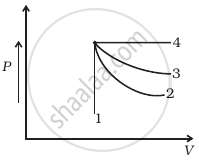• 4

• 3

• 2

• 1

Exercises | Q 12.2 | Page 83

If an average person jogs, hse produces 14.5 × 103 cal/min. This is removed by the evaporation of sweat. The amount of sweat evaporated per minute (assuming 1 kg requires 580 × 103 cal for evaparation) is ______.

• 0.25 kg

• 2.25 kg

• 0.05 kg

• 0.20 kg

Exercises | Q 12.3 | Page 84

Consider P-V diagram for an ideal gas shown in figure.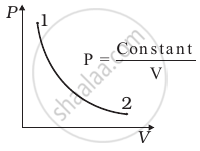Out of the following diagrams (figure), which represents the T-P diagram?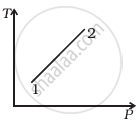(i)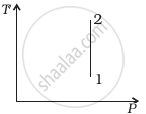(ii)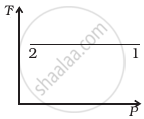(iii)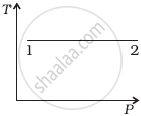(iv)
• (iv)

• (ii)

• (iii)

• (i)

Exercises | Q 12.4 | Page 84

An ideal gas undergoes cyclic process ABCDA as shown in given P-V diagram (figure). The amount of work done by the gas is ______.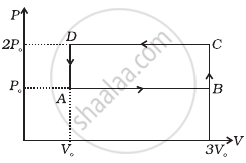• 6P0V0

• –2P0V0

• + 2P0V0

• + 4P0V0

Exercises | Q 12.5 | Page 85

Consider two containers A and B containing identical gases at the same pressure, volume and temperature. The gas in container A is compressed to half of its original volume isothermally while the gas in container B is compressed to half of its original value adiabatically. The ratio of final pressure of gas in B to that of gas in A is ______.

• 2^(γ - 1)

• (1/2)^(γ - 1)

• (1/(1 - γ))^2

• (1/(γ - 1))^2

Exercises | Q 12.6 | Page 85

Three copper blocks of masses M1, M2 and M3 kg respectively are brought into thermal contact till they reach equilibrium. Before contact, they were at T1, T2, T3 (T1 > T2 > T3). Assuming there is no heat loss to the surroundings, the equilibrium temprature T is (s is specific heat of copper)

• T = (T_1 + T_2 + T_3)/3

• T = (M_1T_1 + M_2T_2 + M_3T_3)/(M_1 + M_2 + M_3)

• T = (M_1T_1 + M_2T_2 + M_3T_3)/(3(M_1 + M_2 + M_3))

• T = (M_1T_1s + M_2T_2s + M_3T_3s)/(M_1 + M_2 + M_3)

#### MCQ II

Exercises | Q 12.7 | Page 85

Which of the processes described below are irreversible?

1. The increase in temprature of an iron rod by hammering it.
2. A gas in a small cantainer at a temprature T1 is brought in contact with a big reservoir at a higher temprature T2 which increases the temprature of the gas.
3. A quasi-static isothermal expansion of an ideal gas in cylinder fitted with a frictionless piston.
4. An ideal gas is enclosed in a piston cylinder arrangement with adiabatic walls. A weight W is added to the piston, resulting in compression of gas.
Exercises | Q 12.8 | Page 86

An ideal gas undergoes isothermal process from some initial state i to final state f. Choose the correct alternatives.

1. dU = 0
2. dQ= 0
3. dQ = dU
4. dQ = dW
Exercises | Q 12.9 | Page 86

Figure shows the P-V diagram of an ideal gas undergoing a change of state from A to B. Four different parts I, II, III and IV as shown in the figure may lead to the same change of state.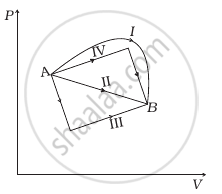1. Change in internal energy is same in IV and III cases, but not in I and II.
2. Change in internal energy is same in all the four cases.
3. Work done is maximum in case I
4. Work done is minimum in case II.
Exercises | Q 12.10 | Page 86

Consider a cycle followed by an engine (Figure)

1 to 2 is isothermal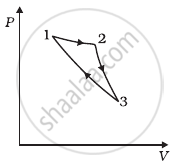Such a process does not exist because ______.

1. heat is completely converted to mechanical energy in such a process, which is not possible.
2. mechanical energy is completely converted to heat in this process, which is not possible.
3. curves representing two adiabatic processes don’t intersect.
4. curves representing an adiabatic process and an isothermal process don’t intersect.
Exercises | Q 12.11 | Page 86

Consider a heat engine as shown in figure Q1 and Q2 are heat added to heat bath T1 and heat taken from T2 in one cycle of engine. W is the mechanical work done on the engine. If W > 0, then possibilities are ______.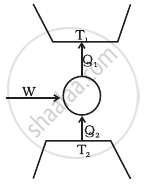1. Q1 > Q2 > 0
2. Q2 > Q1 > 0
3. Q2 < Q1 < 0
4. Q1 < 0, Q2 > 0

#### VSA

Exercises | Q 12.12 | Page 87

Can a system be heated and its temperature remains constant?

Exercises | Q 12.13 | Page 87

A system goes from P to Q by two different paths in the P-V diagram as shown in figure. Heat given to the system in path 1 is 1000 J. The work done by the system along path 1 is more than path 2 by 100 J. What is the heat exchanged by the system in path 2?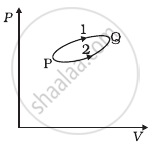Exercises | Q 12.14 | Page 87

If a refrigerator’s door is kept open, will the room become cool or hot? Explain.

Exercises | Q 12.15 | Page 87

Is it possible to increase the temperature of a gas without adding heat to it? Explain.

Exercises | Q 12.16 | Page 87

Air pressure in a car tyre increases during driving. Explain.

#### SA

Exercises | Q 12.17 | Page 87

Consider a Carnot’s cycle operating between T1 = 500 K and T2 = 300 K producing 1 k J of mechanical work per cycle. Find the heat transferred to the engine by the reservoirs.

Exercises | Q 12.18 | Page 87

A person of mass 60 kg wants to lose 5kg by going up and down a 10 m high stairs. Assume he burns twice as much fat while going up than coming down. If 1 kg of fat is burnt on expending 7000 kilo calories, how many times must he go up and down to reduce his weight by 5 kg?

Exercises | Q 12.19 | Page 87

Consider a cycle tyre being filled with air by a pump. Let V be the volume of the tyre (fixed) and at each stroke of the pump ∆V(V) of air is transferred to the tube adiabatically. What is the work done when the pressure in the tube is increased from P1 to P2?

Exercises | Q 12.20 | Page 87

In a refrigerator one removes heat from a lower temperature and deposits to the surroundings at a higher temperature. In this process, mechanical work has to be done, which is provided by an electric motor. If the motor is of 1 kW power, and heat is transferred from – 3°C to 27°C, find the heat taken out of the refrigerator per second assuming its efficiency is 50% of a perfect engine.

Exercises | Q 12.21 | Page 88

If the co-efficient of performance of a refrigerator is 5 and operates at the room temperature (27°C), find the temperature inside the refrigerator.

Exercises | Q 12.22 | Page 88

The initial state of a certain gas is (Pi, Vi, Ti). It undergoes expansion till its volume becomes Vf. Consider the following two cases:

1. the expansion takes place at constant temperature.
2. the expansion takes place at constant pressure.

Plot the P-V diagram for each case. In which of the two cases, is the work done by the gas more?

#### LA

Exercises | Q 12.23 | Page 88

Consider a P-V diagram in which the path followed by one mole of perfect gas in a cylindrical container is shown in figure.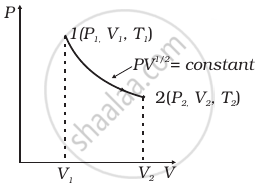1. Find the work done when the gas is taken from state 1 to state 2.
2. What is the ratio of temperature T1/T2, if V2 = 2V1?
3. Given the internal energy for one mole of gas at temperature T is (3/2) RT, find the heat supplied to the gas when it is taken from state 1 to 2, with V2 = 2V1.
Exercises | Q 12.24 | Page 88

A cycle followed by an engine (made of one mole of perfect gas in a cylinder with a piston) is shown in figure.

A to B : volume constant
C to D : volume constant

VC = VD = 2VA = 2VB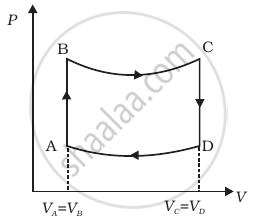1. In which part of the cycle heat is supplied to the engine from outside?
2. In which part of the cycle heat is being given to the surrounding by the engine?
3. What is the work done by the engine in one cycle? Write your answer in term of PA, PB, VA.
4. What is the efficiency of the engine?

(γ = 5/3 for the gas), (Cv = 3/2 R for one mole)

Exercises | Q 12.25 | Page 89

A cycle followed by an engine (made of one mole of an ideal gas in a cylinder with a piston) is shown in figure. Find heat exchanged by the engine, with the surroundings for each section of the cycle. (Cv = (3/2)R)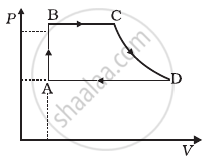1. AB : constant volume
2. BC : constant pressure
4. DA : constant pressure
Exercises | Q 12.26 | Page 89

Consider that an ideal gas (n moles) is expanding in a process given by P = f(V), which passes through a point (V0, P0). Show that the gas is absorbing heat at (P0, V0) if the slope of the curve P = f(V) is larger than the slope of the adiabat passing through (P0, V0).

Exercises | Q 12.27 | Page 89

Consider one mole of perfect gas in a cylinder of unit cross section with a piston attached (figure). A spring (spring constant k) is attached (unstretched length L) to the piston and to the bottom of the cylinder. Initially the spring is unstretched and the gas is in equilibrium. A certain amount of heat Q is supplied to the gas causing an increase of volume from V0 to V1.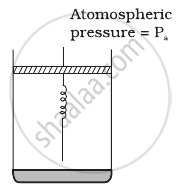1. What is the initial pressure of the system?
2. What is the final pressure of the system?
3. Using the first law of thermodynamics, write down a relation between Q, Pa, V, Vo and k.

## Solutions for Chapter 12: Thermodynamics

Exercises## NCERT Exemplar solutions for Class 11 Physics chapter 12 - Thermodynamics

Shaalaa.com has the CBSE Mathematics Class 11 Physics CBSE solutions in a manner that help students grasp basic concepts better and faster. The detailed, step-by-step solutions will help you understand the concepts better and clarify any confusion. NCERT Exemplar solutions for Mathematics Class 11 Physics CBSE 12 (Thermodynamics) include all questions with answers and detailed explanations. This will clear students' doubts about questions and improve their application skills while preparing for board exams.

Further, we at Shaalaa.com provide such solutions so students can prepare for written exams. NCERT Exemplar textbook solutions can be a core help for self-study and provide excellent self-help guidance for students.

Concepts covered in Class 11 Physics chapter 12 Thermodynamics are Isothermal Processes, Adiabatic Processes, Thermal Equilibrium, Zeroth Law of Thermodynamics, Heat, Internal Energy and Work, First Law of Thermodynamics, Thermodynamic State Variables and Equation of State, Specific Heat Capacity, Thermodynamic Process, Heat Engine, Refrigerators and Heat Pumps, Second Law of Thermodynamics, Reversible and Irreversible Processes, Carnot Engine.

Using NCERT Exemplar Class 11 Physics solutions Thermodynamics exercise by students is an easy way to prepare for the exams, as they involve solutions arranged chapter-wise and also page-wise. The questions involved in NCERT Exemplar Solutions are essential questions that can be asked in the final exam. Maximum CBSE Class 11 Physics students prefer NCERT Exemplar Textbook Solutions to score more in exams.

Get the free view of Chapter 12, Thermodynamics Class 11 Physics additional questions for Mathematics Class 11 Physics CBSE, and you can use Shaalaa.com to keep it handy for your exam preparation.